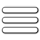ATP EducationThe Secure way of Learning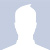Welcome! Guest

Sponser's Link
Sponser's Link

Sponser's Link

CBSE And NCERT Solutions:

Page 3 of 5

# Balancing a Chemical Equation.

Here we would learn the method how to balance a chemical reaction.0

The method which we apply e.i hit and trail or inspecting method. Here we take Equation Fe + H2O → Fe3O4 + H2 as example I:

Steps;

(i)  Write each formula in box or imagine that each formula is in box. It is so that       you have to mind no changes should be made inside the box.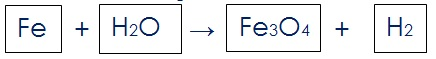(ii)  Count and list the numbers of atom of different element that present in                 unbalanced equation. Like this

Elements              Reactant                               Product

Fe                          1                                              3

O                           1                                              4

H                            2                                              2

First observe that what element has the maximum number of atoms. It may be from reactant and from product. Using this criteria we find that Fe3O4  is that compound and element is O that have maximum 4 atoms.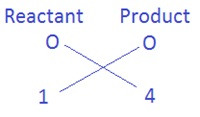To equalise the number of atoms of oxygen, we can put the coefficient 4 with H2O as 4H2O. Then we get the equation as,

Fe + 4H2O → Fe3O4 + H2

(iii)  The next maximum number of atoms having Fe. Which can be equalised

by same process.Putting the coefficient 3 with Fe as 3Fe in reactant side. Now, the equation    will be

3Fe + 4H2O → Fe3O4 + H2

(iv)  Finally we come to equalise Hydrogen atom of both side. We observe that          4H2 in reactant and H2 in product. Total atom of hydrogen in reactant side           is 4 × 2 = 8 and 2 in product. Then,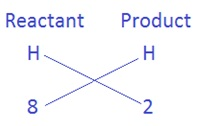Here we cannot put 2 on LHS and 8 on RHS. (8 ÷ 2 ) =4 , the coefficient will be 4 that equalises hydrogen atom on RHS. So finally equation will be.

3Fe + 4H2O → Fe3O4 + 4H2        (balanced equation)

Example II. We take,

HNO3 + Ca(OH)2 → Ca(NO3)2 + H2O

Steps:

(i)  On observing the above chemical equation we see NO3 has maximum number of atoms/molecules. To equalise it we have on LHS 1 molecule and on RHS 2 molecules. So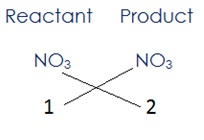Here Nitrogen and oxygen have been balanced, then Equation will be;

2HNO3 + Ca(OH)2 → Ca(NO3)2 + H2O

(ii)  Next calcium is already balanced, now we have to balance only hydrogen atoms.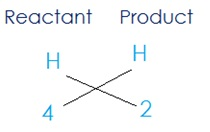Product need 2 as coefficient because (4 ÷ 2) = 2 , then equation will be;

2HNO3 + Ca (OH)2 → Ca(NO3)2 +2H2O

(iii)  On counting and listing the numbers of atom of different element that present in equation above.

Elements              Reactant                   Product

O                           8                                  8

N                           2                                  2

Ca                         1                                  1

H                            4                                  4

Hence we get a balanced equation

2HNO3 + Ca (OH)2 → Ca(NO3)2 +2H2O

I think now you have learnt how to balance a skeletal equation.

www.atpeducation.com

www.atpeducation.com

Advertisement
Page 3 of 5

## Topic Lists:

##### CBSE Notes⇒Class 10th ⇒ Science ⇒ Chapter 1. Chemical Reactions and Equations:

See other sub-topics of this chapter:

Download Our Android AppFeed BackRoshan Class X says: "6"Shadab Khan Class X says: "make fast all science pages geography "Shivam Bajpai All Class says: "ये पेज under construction क्युं है .plz fix this prob..."Krishan Class X says: "this very good website i really appreciate which provide no cost education to all medium classes"Vimal Class XI says: "Not able to find the content....as instructed."Kamini Class X says: "every chepter is imcomplete....this side is not useful"Ashok Swami All Class says: "10th science ka Lesson-8 ka page no.5 kab take under construction rahega please improve it."Rishabh Gupta Class XI says: "how can i understand difference between permutation and combination word problem"Rishabh Gupta Class XI says: "i want to learn parts of speech"KASHIF ALI Class VIII says: "Please update all the syllabus of class 8"
ATP Education

Follow us On Google+
Join Us On Facebook
Sponser's Link
Sponser's Link